# Hill of Banchory geothermal energy project: feasibility report

Report of a study which explored the potential for a deep geothermal heat project at the Hill of Banchory, Aberdeenshire.

### 11. Integrating the Upstream and Downstream Economic Models

We now draw together the analysis set out in the two previous chapters to answer the key question: given the configuration of the current and future heat network at Hill of Banchory, and the financial constraints under which it operates, could it operate using the heat output of the proposed geothermal well, given our estimates of its likely capacity?

In terms of unit heat price, we can summarise our analysis in Figure 30 below, which shows how the unit price of heat sold from the geothermal well (blue line) and the required purchase price of heat for the heat network (green line) change as demand from the heat network increases.

In other words, the blue line indicates the unit price that heat can be sold from the geothermal well and still remain profitable for a given level of investment return. (As explained in the box in section 10, the high up-front capital cost and low operating costs of geothermal heat mean that this price falls steadily as more heat is sold.)

Figure 30: Indicative Cost of Heat Model Outputs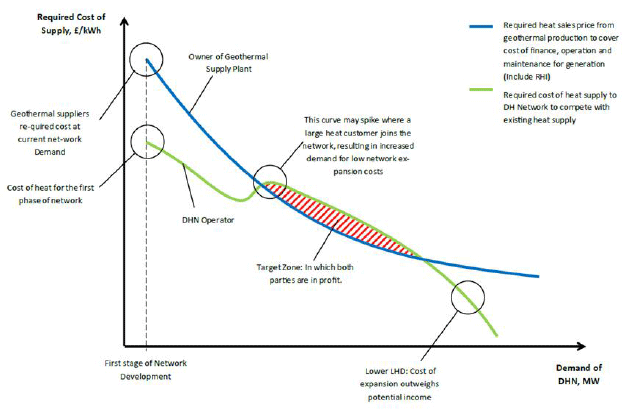Image: Ramboll Energy

The green line indicates the required cost of heat from the point of view of the heat network operator. As the heat network expands to service less densely grouped heat demands, more piping must be installed per unit of heat sold. This means that the network operator must produce heat at a lower unit cost if it is to remain profitable. Although the green line tends to fall from left to right as the size of the network increases, it does not do so monotonically - spikes can appear in the line as large heat customers join the network.

The red shaded area in this (indicative) graph shows the area in which the green line is above the blue line - which means there are levels of heat demand where both the geothermal well and the heat network can make a profit: in other words, the system is economically viable. Does such a region exist for the Hill of Banchory heat network?

Geothermal Heat Cost

As we have seen from Chapter 10, if the geothermal potential of the Hill of Fare site is able to support a geothermal well with a heat capacity of at least a few megawatts, and depending on a sufficient level of heat sales (perhaps 10,000 megawatt-hours per year or higher) it can achieve economic viability with sales of around 2p/kilowatt hour. With higher heat sales, the price could be lowered further and in ideal circumstances could approach 1p per kilowatt hour.

Figure 31: Required heat sales for the geothermal well to be financially viable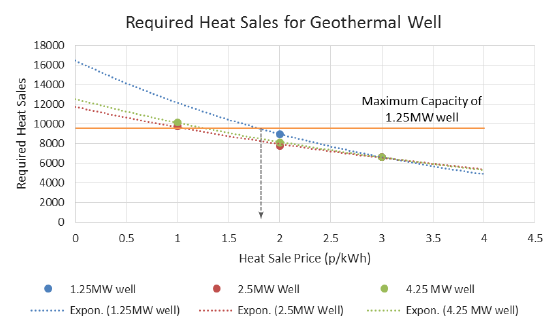The calculations around these results assumed that regardless of heating capacity the well's annual production would be limited to account for low summer demand and periods of maintenance down-time in which it could not run. This resulted in a yearly production limit for the wells calculated from: (well capacity x operating hours) which in turn defined a minimum heat sale price.

The existing HOB network uses biomass fuel. For the geothermal resource to be utilised in the existing HOB network, it would either have to replace the biomass boilers as the main heat supply, or there would have to be enough extra demand from the proposed expansions that it could be included as an extra primary heat source. For the former to be the case, the cost of heat from the geothermal supply would hence have to be lower than the current cost of heat from the biomass boilers. To make this comparison data were obtained from both the current network operator, HOBESCO and the team member responsible for the well costing; Cluff Geothermal.

Current Fuel Cost Benchmark

The cost of delivered wood chips (at 40% moisture content) varies depending on the local market, and is typically around 3.1p/kWh. In comparison, due to their integrated woodfuel supply chain, HOBESCO's cost of biomass fuel is just 1.5p/kWh.

RHI Tariff Considerations

A geothermal project is likely to take several years before it can supply heat. In Banchory further extensions to the HOB network would have been completed and the second biomass boiler would be operational. This would bring the energy centres total capacity to 1.6MW putting it over the "medium commercial scale" threshold of 1MW, and therefore lowering the available RHI income from the second boiler, as shown in Figure 32 below.

Figure 32: Commercial RHI Biomass Tariffs as of January 2016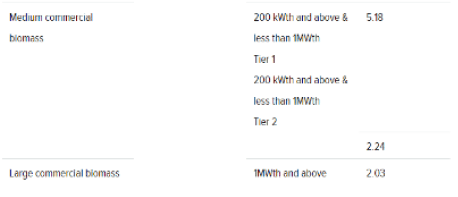A simple comparison of these figures shows that even for the reduced RHI tariff, currently 2.24 p/kWh, HOBESCO has a negative fuel cost of -0.53p/kWh. It should be noted that RHI is only payable on delivered heat, therefore network heat losses would diminish this margin. As well as this, there will be other costs involved in handling the biomass and running the energy centre, but many of these would remain irrespective of the source of heat. Despite these simplifications, the comparison shows that the geothermal supply would have to supply heat to the network at close to zero to be competitive, therefore relying solely on RHI payments for income; currently 5.08p/kWh.

Due to the difficulty of competing with the low cost of heat generation from biomass at Hill of Banchory, geothermal heat supply would have to be at a point where the biomass boilers had reached their maximum generation capacity, when the alternative heat supply would be natural gas. For this analysis the cost of natural gas heating was taken as 3.2p/kWh, based on an 80% efficiency and the most recent DECC announcements available at the time of writing. This suggests that by 2019 (given an assumption of rising fossil fuel prices), geothermal energy would be a potentially favourable option. The next step was therefore to assess the demand growth planned for the network and determine at what point this might be feasible.

Network Growth Projections

To establish how much additional heat supply might be required due to the network expansions, it was first necessary to determine the annual capacity of the existing boilers. This was done by assuming that at their maximum operating conditions the boilers would be running for 5,000 equivalent full load running hours, which was then simply multiplied by the combined boiler capacity (1.6MW).

Figure 33: Demand projections for the Hill of Banchory Heat Network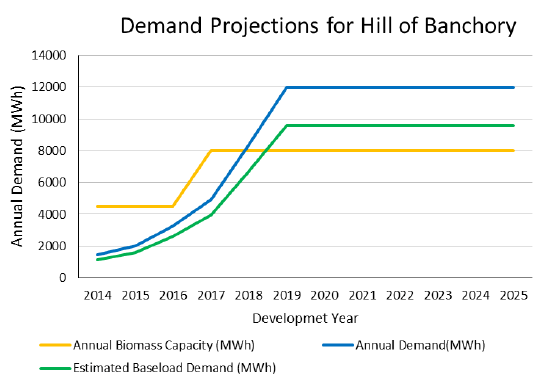Figure 33 shows how the demand on the HOB network is planned to increase over the next 5-10 years along with the estimated generation capacity of the biomass boilers and the estimated proportion of demand that could be supplied by a baseload heat source, namely a geothermal supply. It can be seen that until 2018-19, the biomass boilers can supply the majority of demand, with a deficit of 2,000MWh per annum thereafter.

Initial Conclusions

Considering the cheaper cost of heat that the biomass boilers provide, their maximal operation would be the first priority for HOBESCO, leaving 4,000 MWh per annum of demand that could in theory come from the geothermal supply. Referring back to the heat sales price estimates however, suggests that even when natural gas was the fuel source to compete against, therefore allowing the geothermal heat sale price to be at least 3p/kWh, around 6,500MWh of heat would have to be sold per year for the well to achieve its required IRR, i.e. more demand than is forecast under current plans, meaning the inclusion of at least some of the networks described in Chapter 9.

The Network's required cost of heat

Our analysis suggests that for a geothermal heat scheme to have sufficient heat sales in Banchory to be commercially viable, extra demand above the existing forecast for the Hill of Banchory Network is required. A model was built to calculate how the cost of heat varies at different geographical scales of network and capacity of supply asset, using data from HOBESCO for the existing network and heat map data from the Scottish Government for the wider network opportunities (a list of the input variables is found in Appendix 2). The calculated cost of heat from the geothermal plant is shown in Figure 34.

Figure 34: Cost of heat model cumulative expansion results for proposed Banchory networks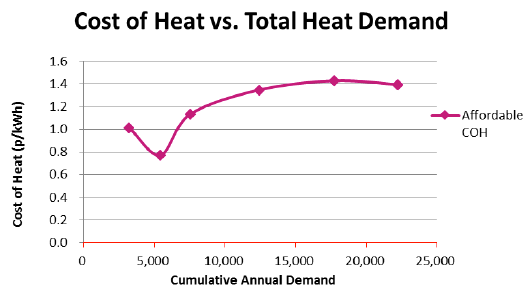Figure 34 shows that for most scales of network, the Banchory scheme could afford to pay at least 1p/kWh for its heat, a figure that is achievable from the geothermal operator's point of view provided at least 10 GWh of heat were sold per year.